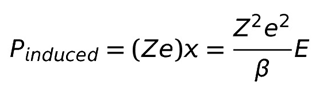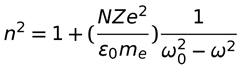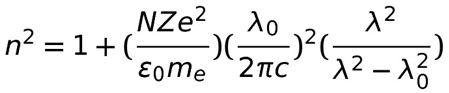# Dispersion Relation

In Figure 1, an incident train of light pulses strikes the surface of the transparent prism. At the surface of the prism, each wavelength of the pulse separates into its constituent light rays (red, yellow, blue, etc.). The separation is due to the material index of refraction, n, changing with wavelength. The index of refraction is the ratio of the phase velocity outside to inside the material. In vacuum, light’s speed is c, and inside the prism, it is v, so n = c/v and varies with wavelength, λ. This variation causes a separation in the phase velocity and direction of each colored wavelength, causing them to separate. This separation is called dispersion. In other words, dispersion is the change of the index of refraction of a material as a function of the wavelength of light that is traveling through the material . The dispersion relation relates the index of refraction of a material to a wavelength of light traveling through the material.

## Background## Induced electronic dipole moment

In Figure 2, an atom is undisturbed, as it is not in an applied electric field. Because of this, the center of mass of its electron cloud is centered on its nucleus with perfect spherical symmetry. Things get interesting when an electric field is applied. In Figure 3, an electric field, E, known as a wave of light (see electromagnetic radiation, enters a material and attracts the electron clouds of the atoms in the material. This attraction of the electron cloud by the electric field (light wave) causes the center of mass of the electron cloud to move x distance away from the origin (center of the nucleus) and toward the electric field.

This electric field pulls the electrons toward it with a force of ZeE where Z is the effective nuclear charge (very roughly, the number of protons per atom), e is the charge of an electron, and E is the magnitude of the applied electric field. This force of the applied field is counteracted by the restoring force, shown as Frestoring in Figure 3, which is caused by the attraction of the electron cloud to the nucleus. The restoring force can be written as Frestoring= -βx where β is a constant and x is, again, the distance between the origin and center of mass of the electron cloud.

In the case where an direct current electric field is suddenly applied to the atom, the electron cloud begins moving as it responds to and shifts toward the field. Now, after enough time has passed, the system equilibrates, and the electron cloud is now motionless. In this case, the induced dipole moment’s magnitude isZ is the effective nuclear charge, e is the charge of an electron, x is the distance of the center of mass of the electron cloud from the nucleus, β is a constant, and E is the magnitude of the applied electric field.

Now, if the applied electric field is turned off, then the only force acting on the electron cloud is the restoring force. Frestoring= -βx = mass*acceleration:where me is the mass of an electron. Recall Z is not just the number of protons but also the number of electrons, so Zme is the total mass of all electrons in an atom. 

## Simple harmonic oscillation and resonance frequency

Solving the previous equation for x yieldsThis equation shows the electron cloud undergoes simple harmonic oscillation at the resonance frequency, ωo, when the electric field is removed. ωo is given byFigure $$\PageIndex{3}$$: , the electromagnetic wave that passes through the atom has an oscillating electric field given as E = Eoexp(jωt). In this equation, Eo is the maximum amplitude of the electromagnetic wave, t is time, and ω is the angular frequency; ω = 2πf where f is the frequency of the electromagnetic wave. This oscillating field produces a force, -ZeEoexp(jωt), that, together with the restoring force, -βx, caused by the nucleus, oscillates the electron cloud around the nucleus. By using Newton’s second law, the Lorentz oscillator model is derived:Solving this equation for x gives## Electronic polarizability and the dielectric constant

Plugging x back into the P_induced equation, dividing by the electric field of the electromagnetic wave, and taking the absolute value gives the magnitude of the electronic polarizability:Recall ω is the angular frequency of the electromagnetic wave, and ωo is the resonance frequency. By inspection, as ω approaches ωo, the electronic polarizability, induced electronic dipole moment, and displacement of the electron cloud should theoretically increase to infinity. However, this is not the case, because this equation is only valid for small displacements of the electron cloud.

A ballpark yet concise relationship between the electronic polarizability and the dielectric constant, εr, isN is atoms per volume, and εo, a constant, is the permittivity of free space. The dielectric constant is related to the index of refraction by n^2 = εr. Thus, substituting the full electronic polarizability equation into the dielectric constant equation gives the dispersion relation that is shown next. 

## Dispersion relation

The dispersion relation isThe dispersion relation can be used to determine n for different wavelengths of electromagnetic radiation passing through a material. For example, if the resonance frequency for a given material is around the frequency of ultraviolet light, then the material will have consecutively larger n values for red, yellow, and blue wavelengths of light, because this is the order in which these wavelengths are closer to the resonance wavelength. 

To better understand the equation, plugging in values is a good idea. As N or Z approaches zero, n approaches 1. This makes sense, because if there are almost zero atoms in a material, then the speed of electromagnetic radiation through it, v, should be close to that in vacuum, c. Hence, because n = c/v, n should be just over unity, which seems reasonable.

The equation does not make physical sense for ω values greater than or equal to ωo. The former case would give n values less than one, which is not possible, because electromagnetic radiation cannot travel faster than the speed of light in vacuum. The latter case gives a discontinuity in the n values, which does not occur from real life measurements. This is reconciled by the fact that a damping term was supposed to go in the denominator of the dispersion relation alongside the angular frequencies but was not, perhaps to simplify the expression .

## Other forms of the dispersion relation

Table A. Sellmeier coefficients and resonant frequencies of materials. Redrawing of Table. 9.2 from: Kasap, S.O. (2006). Principles of Electronic Materials and Devices (3rd ed.). Boston: McGraw-Hill.

Material

A

B

C

λA (nm)

λB (nm)

λC (nm)

Sapphire

1.023798

1.058264

5.280792

61.4482

110.700

17,926.56

Fused Silica

0.696749

0.408218

0.890815

69.0660

115.662

9,900.559

Diamond

0.3306

4.3356

N/A

175.0

106.0

N/A

Table B. Cauchy coefficients of materials. Redrawing of Table. 9.2 from: Kasap, S.O. (2006). Principles of Electronic Materials and Devices (3rd ed.). Boston: McGraw-Hill.

Material

hν range [eV]

n1

[(eV)^2]

n2

[unitless]

n3

[1/(eV)^-2]

n4

[1/(eV)^-4]

Silicon

0.002-1.08

-2.04* 10^-8

3.4189

8.15*10^-2

1.25*10^-2

Diamond

0.05-5.47

-1.07* 10^-5

2.378

8.01*10^-3

1.04*10^-4

The relationships ω = 2πf and f = c/λ can be used to rewrite the dispersion relation:To determine how n varies λ with a crystal, a more complex handling of the dispersion relation is needed, because there can be multiple resonant frequencies (λA, λB, λC, etc.). The Sellmeier-Herzberger formula accounts for this:A, B, and C are Sellmeier coefficients and are given in Table A.

Cauchy developed the first dispersion relation equation . It is simple but only works for a limited range of energy values, as shown in Table B. Cauchy dispersion relation equation:The values for n1, n2, n3, and n4 are given in Table B. When using this equation, a useful relation to have at hand is hν = hc/λ where h, Planck’s constant, is 4.136*10^-15 eV*s, and c, the speed of light in vacuum, is 3.00*10^8 m/s. 

## Questions

1. Using the Cauchy equation, what is the index of refraction of silicon at 1212nm?

2. Using the data in Table B and the Cauchy equation, is it feasible for one to calculate the index of refraction of silicon at optical wavelengths, given that they span from 380nm-750nm; why or why not?

3. Using the Sellmeier-Herzberger formula and Table A, what is the index of refraction of diamond for yellow sodium light, which has a wavelength of 589nm? How does your value compare to the experimental value of n = 2.417 at 589nm for diamond ?

## Solutions

1. hν = hc/λ = (4.136*10^-15 [eV*s])(3.00*10^8 [m/s])/(1212*10^(-9) [m]) = (517/505) [eV]

n = n1(hν)^(-2) + n2 + n3(hν)^(2) + n4(hν)^(4) =

(-2.04*10^(-8) [(eV)^2])((517/505) [eV])^(-2) + 3.4189 + (8.15*10^-2 [1/(eV)^-2])((517/505) [eV])^(2) + (1.25*10^-2 [1/(eV)^4])((517/505) [eV])^(4) = 3.518

2. It is not feasible, because if one calculates the hν value for 750nm (it is simplest to only calculate this value), the result is 1.65 [eV], which is outside the range of hν values for which the Cauchy equation applies; this range is shown in Table B.

3. n^2 = 0.3306*(589*10^-9 [m])^(2)/(((589*10^-9 [m])^2 – (175.0*10^-9 [m])^2 + 4.3356*(589*10^-9 [m])^(2)/(((589*10^-9 [m])^2 – (106.0*10^-9 [m])^2 = 2.417. Keeping in mind that the experimental value was only reported to three significant figures, one may hesitantly say the calculated answer is identical to the experimental value.

## References

1. Hyperphysics, Dispersion, [online] 2004, hyperphysics.phy-astr.gsu.edu...ispersion.html
2. Kasap, S.O. (2006). Principles of Electronic Materials and Devices, Figure 9.4 (3rd ed.). Boston: McGraw-Hill.
3. Kasap, S.O. (2006). Principles of Electronic Materials and Devices, pages 779-784 (3rd ed.). Boston: McGraw-Hill.
4. Hecht, Eugene and Zajac, Alfred. (December 1976). Optics, page 41. (Third printing). Philippines: Addison-Wesley Publishing Company, Inc.
5. Horiba, Cauchy and Related Empirical Dispersion Formulae for Transparent Materials, [online] 2006, www.horiba.com/fileadmin/uplo..._Materials.pdf
6. Young and Freedman (2012). University Physics Volume Two, pg. 1086, (13th edition). San Francisco: Addison-Wesley.